Home > CC4 > Chapter A > Lesson A.1.7 > ProblemA-71

A-71.

Solve the following equations. Record your work and check your answers. Homework Help ✎

1.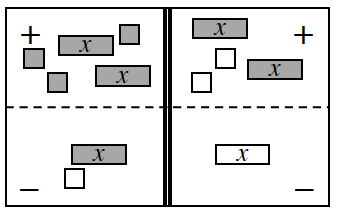2.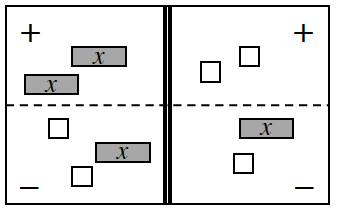3. 3c − 7 = −c + 1

4. −2 + 3x = 2x + 6 + x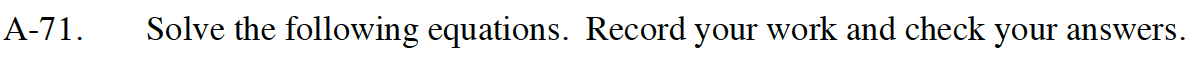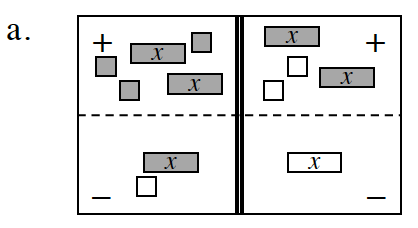The dark boxes are positive, and the white boxes are negative.
Watch out for subtraction errors when completing this problem.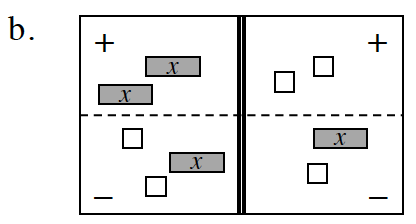The dark boxes are positive, and the white boxes are negative.
Watch out for subtraction errors when completing this problem.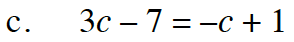Assume that c = x.

c = 2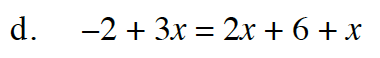no solution